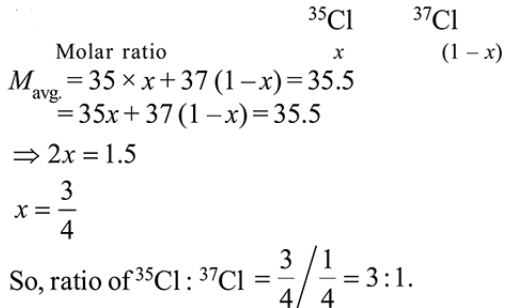# The average molar mass of chlorine isQuestion:

The average molar mass of chlorine is $35.5 \mathrm{~g} \mathrm{~mol}^{-1}$. The ratio of ${ }^{35} \mathrm{Cl}$ to ${ }^{37} \mathrm{Cl}$ in naturally occrring chlorine is close to :

1.  $4: 1$

2. $3: 1$

3. $2: 1$

4. $1: 1$

Correct Option: , 2

Solution: